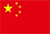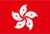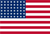Scanning QR Code• Online Inquiry

+
Congratulations
You've successfully got advanced quotes worth 5000 HKD
• Home
• Quotes
• News
• Learn
• Help Center
• Language
•••EN

# EMA index smooth moving average

## 1. Introduction

An exponential moving average (EMA) is a type of moving average (MA) that places a greater weight and significance on the most recent data points. The exponential moving average is also referred to as the exponentially weighted moving average. An exponentially weighted moving average reacts more significantly to recent price changes than a simple moving average (SMA), which applies an equal weight to all observations in the period. EMA5, EMA10, EMA20, EMA60, EMA120, EMA250 correspond to the exponential smoothing moving averages of 5, 10, 20, 60, 120, and 250 respectively.

## 2. Calculation formula

Find the N-day exponential moving average of X, which is generally expressed as: EMA (X, N) in the stock formula. Where X is the closing price of the day and N is the number of days.

Index average value of the day = Smoothing coefficient * (Index value of the day - Yesterday’s index average) + Yesterday’s index average; Smoothing coefficient = 2 / (period unit + 1); Derived from the above formula, we get: EMA (N) = 2 * X / (N+1) + (N-1) * EMA (N-1) / (N+1).

## 3. Application

In actual trading activity, the EMA indicator mainly has the following six basic usages.

● The 5-day EMA and10-day EMA lines is the short-term cycle, the 20-day EMA line is the mid-term cycle, and the 60-day EMA line is the long-term judgment cycle. Among them, the 60-day EMA line is the critical turning point for bull and bear.

● 5-day EMA crossing above the 10-day EMA line is a short-term buy signal; breaking the below the 10-day EMA line is a short-term selling signal.

● 10-day EMA crossing above the 20-day EMA line is a mid-term buy signal; breaking below the 20-day EMA line is a mid-term sell signal.

● 20-day EMA line crossing above the 60-day EMA line is a long-term buy signal; breaking below the 60-day EMA line is a long-term selling signal.

● If the 5-day EMA line crosses or breaks the 20-day EMA line, it will be viewed as a mid to short-term trend; the 10-day EMA line crosses or breaks the 10-day EMA line, it will be viewed as a mid to long-term trend.

● Use EMA to buy the dip and MA to recognize the peak. For example, use the 20-day EMA line to determine the bottom and the 20-day MA line to determine the top.

Back to the Top